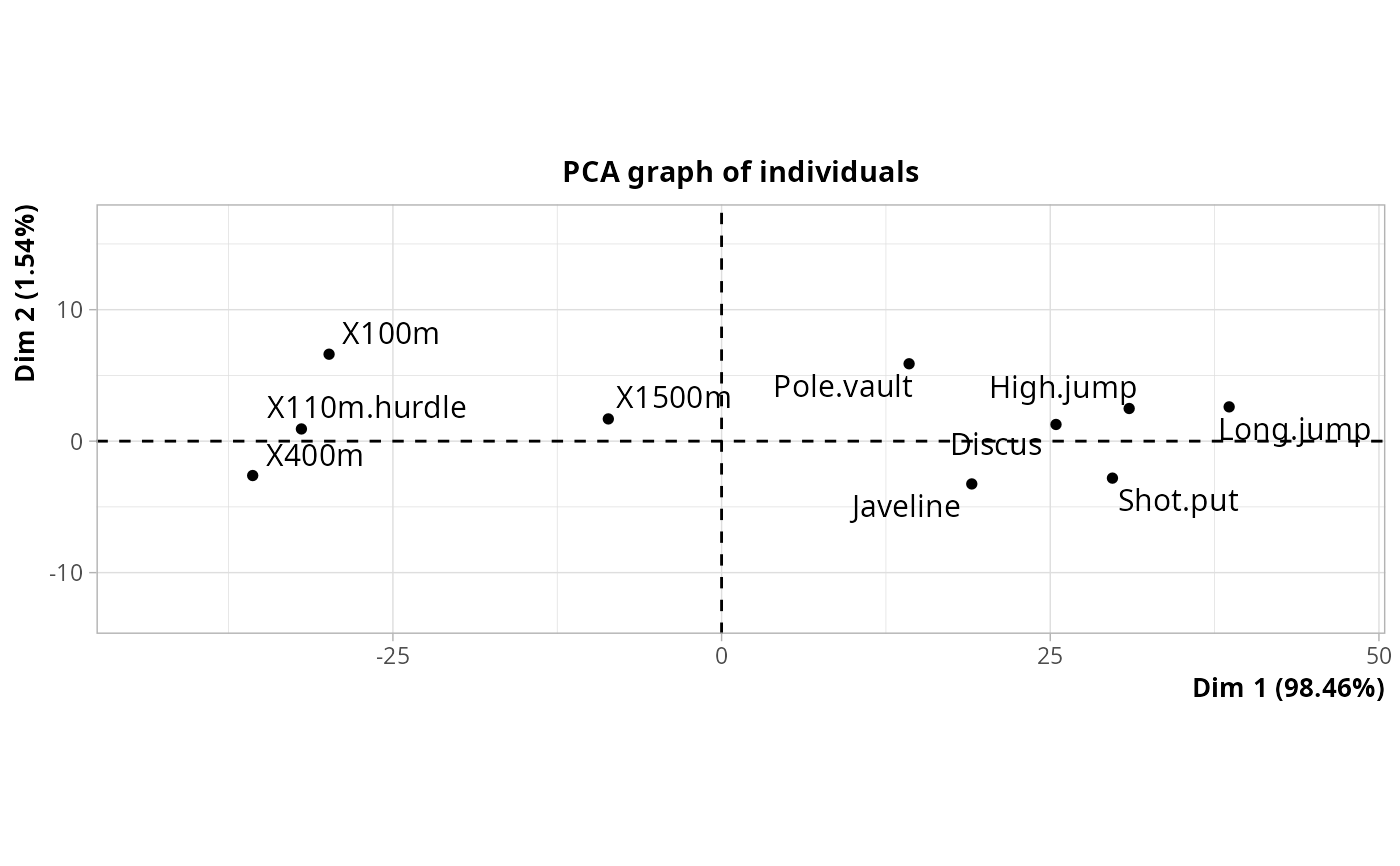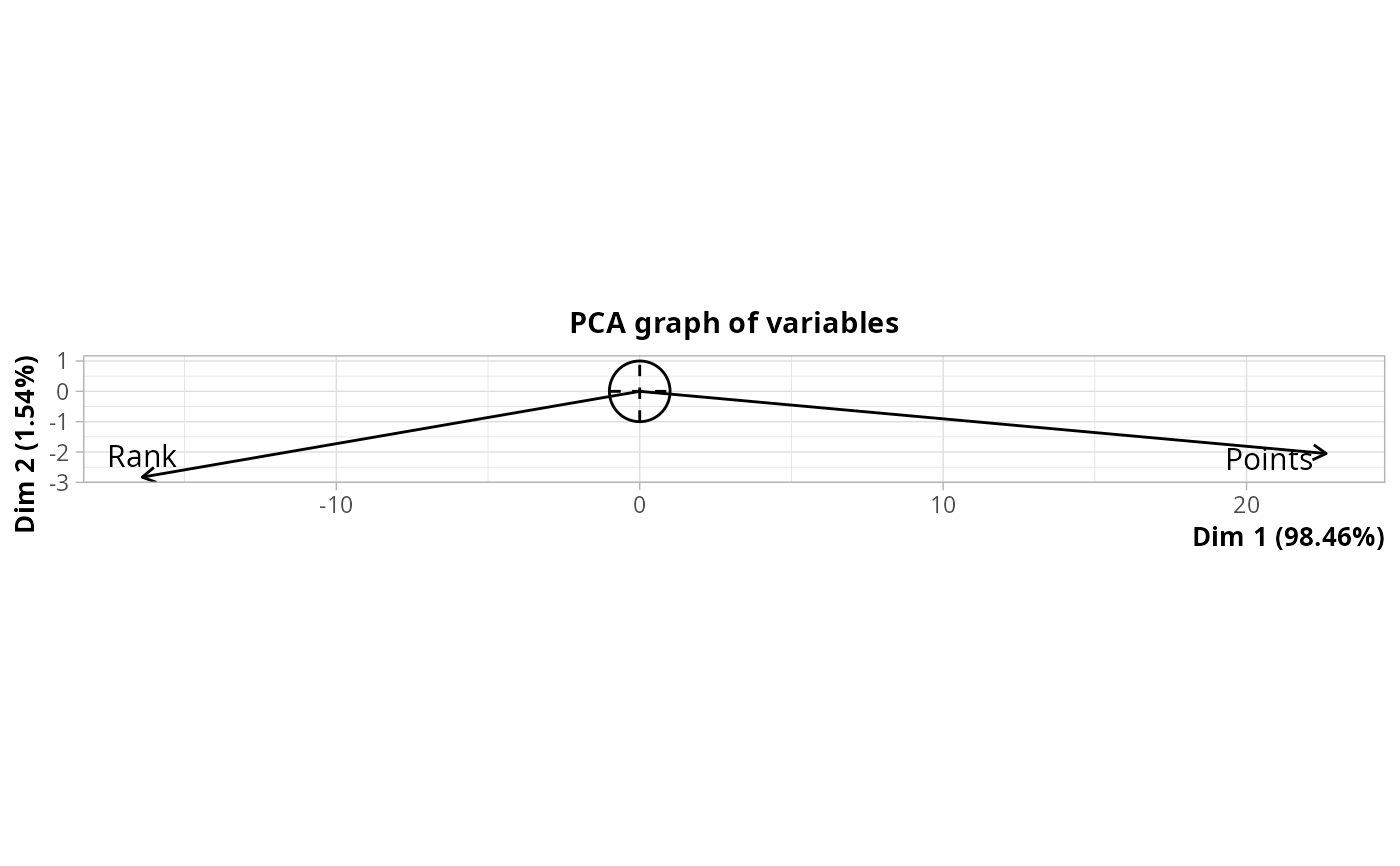Coinertia analysis between two groups of numerical variables

coiPCA(Xa, Xb, row.w = NULL, ncp = 5)

## Arguments

Xa

data frame with the first group of numerical variables

Xb

data frame with the second group of numerical variables

row.w

numeric vector of row weights. If NULL (default), a vector of 1 for uniform row weights is used.

ncp

number of dimensions kept in the results (by default 5)

## Details

Coinertia analysis aims at capturing the structure common to two groups of variables. With groups of numerical variables, it is equivalent to Tucker's inter-battery analysis. It consists in the following steps : 1. Variables in Xa and Xb are centered and scaled 2. Computation of the covariance matrix t(Xa).Xb 3. PCA of the matrix

## Value

An object of class PCA from FactoMineR package, with an additional item :

RV

the RV coefficient between the two groups of variabels

## Author

Nicolas Robette

coiMCA, rvcoef

## Examples

library(FactoMineR)
data(decathlon)
# variables of results for each sport
Xa <- decathlon[,1:10]
# rank and points variables
Xb <- decathlon[,11:12]
# coinertia analysis
res <- coiPCA(Xa, Xb)
# plot of variables in Xa
plot(res, choix = "ind")# plot of variables in Xb
plot(res, choix = "var")# RV coefficient
res\$RV
#>  0.09265003